International
Tables for
Crystallography
Volume F
Crystallography of biological macromolecules
Edited by M. G. Rossmann and E. Arnold

International Tables for Crystallography (2006). Vol. F, ch. 11.1, pp. 209-211   | 1 | 2 |
https://doi.org/10.1107/97809553602060000674

Chapter 11.1. Automatic indexing of oscillation images

M. G. Rossmanna*

aDepartment of Biological Sciences, Purdue University, West Lafayette, IN 47907-1392, USA
Correspondence e-mail: mgr@indiana.bio.purdue.edu

The first step in indexing an oscillation diffraction pattern is to determine the position of diffraction maxima and to record their coordinates on the detector relative to the camera axes. These coordinates can then be transformed into reciprocal-lattice coordinates assuming a zero rotation angle. These positions are then projected onto a vector emanating from the crystal position. If this radius vector is chosen perpendicular to a principal set of reciprocal-lattice planes for the given crystal setting, then a plot of the frequency of the projected reflections onto the vector will show a regular set of equally spaced maxima. These will correspond to the intersection of reciprocal-lattice planes with the selected radius vector. All possible directions, separated from each other by small incremental angles, are tested. One-dimensional Fourier inversion of each of the frequency plots identifies those directions that intersect widely spaced reciprocal-lattice planes, corresponding to principal reciprocal axial directions. Thus the orientation and lattice repeats of the crystal axes will have been determined relative to the camera axes. These parameters can be converted into a 3 × 3 crystal-setting matrix, [A], which relates Miller indices to reciprocal-lattice coordinates.

11.1.1. Introduction

| top | pdf |

Auto-indexing routines have been used extensively for initiating diffraction data collection with a single-point-detector device (Sparks, 1976,1982). These methods depend upon the precise knowledge of the reciprocal-lattice vectors for a few selected reflections. Greater difficulty has been encountered for automatic indexing of oscillation images recorded on two-dimensional detectors using randomly oriented crystals, as is frequently the case for macromolecular crystal samples. In the past, the practice was to orient crystals relative to the camera axes with an accuracy of at least 1°. In this case, the indexing procedure required only refinement of the crystal orientation matrix (Wonacott, 1977; Rossmann, 1979). The American method' (Rossmann & Erickson, 1983), where crystals are oriented more or less randomly, is currently used because of the need for optimizing available synchrotron time and because of the deterioration in radiation-sensitive crystals during the setting process.

A variety of techniques were suggested to determine the crystal orientation, some of which required initial knowledge of the cell dimensions (Vriend & Rossmann, 1987; Kabsch, 1988), while more advanced techniques (Kim, 1989; Higashi, 1990; Kabsch, 1993) determined both cell dimensions and crystal orientation. All these methods start with the determination of the reciprocal-lattice vectors assuming that the oscillation photographs are stills'. The methods of Higashi and Kabsch, as well as, in part, Kim's, analyse the distribution of the difference vectors generated from the reciprocal-lattice vectors. The most frequent difference vectors are taken as the basis vectors defining the reciprocal-lattice unit cell and its orientation. In addition, Kim's technique requires the input of the orientation of a likely zone-axis direction onto which the reciprocal-lattice vectors are then projected. The projections will have a periodicity distribution consistent with the reciprocal-lattice planes perpendicular to the zone axis. Duisenberg (1992) used a similar approach for single-point-detector data, although he did not rely on prior knowledge of the zone-axis direction. Instead, he defined possible zone axes as being perpendicular to a reciprocal-lattice plane by combining three, suitably chosen, reciprocal-lattice points.

None of the above techniques were entirely satisfactory as they sometimes failed to find a suitable crystal orientation matrix. A major advance was made in the program DENZO , a part of the HKL package (Otwinowski & Minor, 1997), which not only has a robust indexing procedure but also has a useful graphical interface. Unfortunately, the indexing technique used in the procedure has never been described, except for a few hints in the manual on the use of an FFT (fast Fourier transform). Indeed, Bricogne (1986) suggested that a three-dimensional Fourier transformation might be a powerful indexing tool, and Strouse (1996) developed such a procedure for single-point-detector data. However, for large unit cells this procedure requires an excessive amount of memory and time (Campbell, 1997).

11.1.2. The crystal orientation matrix

| top | pdf |

The position x (x, y, z) of a reciprocal-lattice point can be given asThe matrix [Φ] is a rotation matrix around the camera's spindle axis for a rotation of ϕ. The vector h represents the Miller indices (h, k, l) and [A] defines the reciprocal unit-cell dimensions and the orientation of the crystal lattice with respect to the camera axes when ϕ = 0. Thus,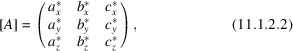whereand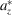are the components of the crystalaxis with respect to the orthogonal camera axes. When an oscillation image is recorded, the position of a reciprocal-lattice point is moved fromto, corresponding to a rotation of the crystal fromto. The recorded position of the reflection on the detector corresponds to the point x when it is on the Ewald sphere somewhere betweenand. The actual value of ϕ at which this crossing occurs cannot be retrieved from the oscillation image. We shall therefore assume here, as is the case in all other procedures, thatdefines the crystal orientation in the centre of the oscillation range. Defining the camera axes as in Rossmann (1979), it is easy to show that a reflection recorded at the position (X, Y) on a flat detector normal to the X-ray beam, at a distance D from the crystal, corresponds to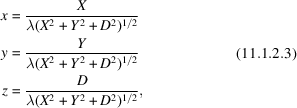where λ is the X-ray wavelength.

If an approximate [A] matrix is available, the Miller indices of an observed peak at (X, Y) can be roughly determined using (11.1.2.3) and (11.1.2.1), where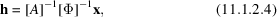with the error being dependent upon the width of the oscillation range, the error in the detector parameters and errors in determining the coordinates of the centres of the recorded reflections.

11.1.3. Fourier analysis of the reciprocal-lattice vector distribution when projected onto a chosen direction

| top | pdf |

If the members of a set of reciprocal-lattice planes perpendicular to a chosen direction are well separated, then the projections of the reciprocal-lattice vectors onto this direction will have an easily recognizable periodic distribution (Fig. 11.1.3.1). Unlike the procedure of Kim (1989), which requires the input of a likely zone-axis direction, the present procedure tests all possible directions and analyses the frequency distribution of the projected reciprocal-lattice vectors in each case. Also, unlike the procedure of Kim, the periodicity is determined using an FFT.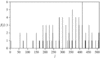Figure 11.1.3.1 | top | pdf |Frequency distribution of the projected reciprocal-lattice vectors for a suitably chosen direction of a diffraction pattern from a fibritin crystal (Tao et al., 1997). Reproduced with permission from Steller et al. (1997).

Let t represent a dimensionless unit vector of a chosen direction. Then, the projection p of a reciprocal-lattice point x onto the chosen vector t is given by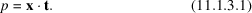To apply a discrete FFT algorithm, all such projections of the reciprocal-lattice points onto the chosen direction t are sampled in small increments of p. For the given direction, the values of the projections are in a range between the endpoints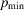and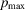. If the maximum real cell dimension is assumed to be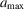, then the maximum number of reciprocal-lattice planes between the observed limits of p is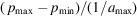. Hence, the number of useful grid points along the direction t should be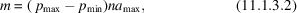where n represents the number of grid points between successive reciprocal-lattice planes and is normally set to 5. Then, the frequency f(p) in the range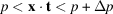can be given as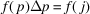, where j is the closest integer to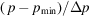and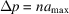. Thus, the discrete Fourier transform of this frequency distribution will be given by the summation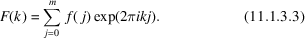The transform is then calculated using a fast Fourier algorithm for all integer values between 0 and m/2 (Fig. 11.1.3.2). The Fourier coefficients that best represent the periodicity of the frequency distribution will be large. The largest coefficient will occur at k = 0 and correspond to the number of vectors used in establishing the frequency distribution. The next set of large coefficients will correspond to the periodicity that represents every reciprocal-lattice plane. The ratio of this maximum to F(0) will be a measure of the tightness of the frequency distribution around each lattice plane. Subsequent maxima will be due to periodicities spanning every second, third etc. frequency maximum and will thus be progressively smaller (Fig. 11.1.3.2). The largest F(k) (when k = l), other than F(0), will, therefore, correspond to an interval of d * between reciprocal-lattice planes in the direction of t where d * = l/(namax).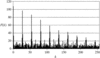Figure 11.1.3.2 | top | pdf |Fourier analysis of the distribution shown in Fig. 11.1.3.1. The first maximum, other than F(0), is at k = 27, corresponding to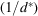= 41.9 Å and a value of F (27) = 97.0. Reproduced with permission from Steller et al. (1997).

11.1.4. Exploring all possible directions to find a good set of basis vectors

| top | pdf |

The polar coordinates ψ, ϕ will be used to define the direction t, where ψ defines the angle between the X-ray beam and the chosen direction t. The Fourier analysis is performed for each direction t in the range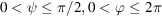. A suitable angular increment in ψ was determined empirically to be about 0.03 rad (1.7°). For each value of ψ, the increment in ϕ is taken to be the closest integral value to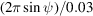. This procedure results in ~7300 separate, roughly equally spaced, directions.

For each direction t, the distribution of the corresponding F(k) coefficients is surveyed to locate the largest local maximum at k = l. The ψ and ϕ values associated with the 30 largest maxima are selected for refinement by a local search procedure to obtain an accuracy of 10−4 rad (~0.006°). If the initial angular increment (0.03 rad) used for the hemisphere search was reduced, then it would not be necessary to refine quite as many local maxima. However, to increase the efficiency of the search procedure, the ratio of angular increments to the number of refined positions was chosen to minimize the total computing time. The F (l) values of the refined positions are then sorted by size. Directions are chosen from these vectors to give a linearly independent set of three basis vectors of a primitive real-space unit cell. These are then converted to the basis vectors of the reciprocal cell. The components of the three reciprocal-cell axes along the three camera axes are the nine components of the crystal orientation matrix [A] (11.1.2.2).

The final step in the selection of the best [A] matrix is to choose various nonlinear combinations of the refined vectors that have the biggest F (l) values. That set of three vectors which gives the best indexing results is then chosen to represent the crystal orientation matrix [A]. A useful criterion is to determine the nonintegral Miller indices h′ from (11.1.2.4) using the [A] matrix and the known reciprocal-lattice vectors x. Any reflection for which any component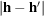is bigger than, say, 0.2 is rejected. The best [A] matrix is chosen as the one with the least number of rejections. In most cases, the best combination corresponds to taking the three largest F (l) values.

The program goes on to determine a reduced cell from the cell obtained by the above indexing procedure (Kim, 1989). The reduced cell is then analysed in terms of the 44 lattice characters (Burzlaff et al., 2005; Kabsch, 1993) in order to evaluate the most likely Bravais lattice and crystal system.

11.1.5. The program

| top | pdf |

The auto-indexing program has been written in C and implemented on an SGI O2 workstation. It is a component of the general data-processing system (DPS ). The auto-indexing component of DPS is available over the web, including the source code. The run-time for auto-indexing is sufficiently short for the procedure to be run interactively. The program described here is now included in the MOSFLM data-processing package (Leslie, 1992).

Acknowledgements

This short article is adapted from a fuller description of the auto-indexing procedure (Steller et al., 1997). I thank Cheryl Towell and Sharon Wilder for help in the preparation of this manuscript. The work was supported by a grant from the National Science Foundation to MGR. Ingo Steller, who wrote and developed the program, was supported by a Deutsche Forschungsgemeinschaft postdoctoral fellowship.

References

Bricogne, G. (1986). Indexing and the Fourier transform. In Proceedings of the EEC cooperative workshop on position-sensitive detector software (phase III), p. 28. LURE, Paris, 12–19 November.Google Scholar
Burzlaff, H., Gruber, B., Zimmermann, H. & de Wolff, P. M. (2005). Crystal lattices. In International tables for crystallography, Vol. A. Space-group symmetry, edited by Th. Hahn, Part 9. Heidelberg: Springer.Google Scholar
Campbell, J. W. (1997). In CCP4 Newsletter, No. 33, edited by M. Winn. Warrington: Daresbury Laboratory.Google Scholar
Duisenberg, A. J. M. (1992). Indexing in single-crystal diffractometry with an obstinate list of reflections. J. Appl. Cryst. 25, 92–96.Google Scholar
Higashi, T. (1990). Auto-indexing of oscillation images. J. Appl. Cryst. 23, 253–257.Google Scholar
Kabsch, W. (1988). Automatic indexing of rotation diffraction patterns. J. Appl. Cryst. 21, 67–71.Google Scholar
Kabsch, W. (1993). Automatic processing of rotation diffraction data from crystals of initially unknown symmetry and cell constants. J. Appl. Cryst. 26, 795–800.Google Scholar
Kim, S. (1989). Auto-indexing oscillation photographs. J. Appl. Cryst. 22, 53–60.Google Scholar
Leslie, A. G. W. (1992). Recent changes to the MOSFLM package for processing film and image plate data. In CCP4 and ESF-EACMB Newsletter on Protein Crystallography, No. 26. Warrington: Daresbury Laboratory.Google Scholar
Otwinowski, Z. & Minor, W. (1997). Processing of X-ray diffraction data collected in oscillation mode. Methods Enzymol. 276, 307–326.Google Scholar
Rossmann, M. G. (1979). Processing oscillation diffraction data for very large unit cells with an automatic convolution technique and profile fitting. J. Appl. Cryst. 12, 225–238.Google Scholar
Rossmann, M. G. & Erickson, J. W. (1983). Oscillation photography of radiation-sensitive crystals using a synchrotron source. J. Appl. Cryst. 16, 629–636.Google Scholar
Sparks, R. A. (1976). Trends in minicomputer hardware and software. Part I. In Crystallographic computing techniques, edited by F. R. Ahmed, K. Huml & B. Sedláček, pp. 452–467. Copenhagen: Munksgaard.Google Scholar
Sparks, R. A. (1982). Data collection with diffractometers. In Computational crystallography, edited by D. Sayre, pp. 1–18. New York: Oxford University Press.Google Scholar
Steller, I., Bolotovsky, R. & Rossmann, M. G. (1997). An algorithm for automatic indexing of oscillation images using Fourier analysis. J. Appl. Cryst. 30, 1036–1040.Google Scholar
Strouse, C. E. (1996). Personal communication.Google Scholar
Tao, Y., Strelkov, S. V., Mesyanzhinov, V. V. & Rossmann, M. G. (1997). Structure of bacteriophage T4 fibritin: a segmented coiled coil and the role of the C-terminal domain. Structure, 5, 789–798.Google Scholar
Vriend, G. & Rossmann, M. G. (1987). Determination of the orientation of a randomly placed crystal from a single oscillation photograph. J. Appl. Cryst. 20, 338–343.Google Scholar
Wonacott, A. J. (1977). Geometry of the rotation method. In The rotation method in crystallography, edited by U. W. Arndt & A. J. Wonacott, pp. 75–103. Amsterdam: North-Holland Publishing Co.Google Scholar